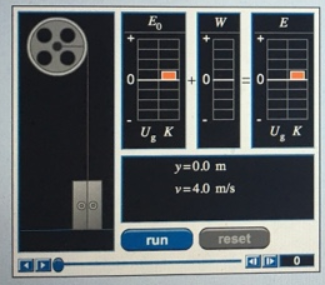# Problem: Look at this applet. It shows an elevator with a small initial upward velocity being raised by a cable. The tension in the cable is constant. The energy bar graphs are marked in intervals of 600 J. Part A What is the mass m of the elevator? Use g=10m/s2 for the magnitude of the acceleration of gravity. Express your answer in kilograms to two significant figures.

###### FREE Expert Solution

From potential energy, U  = mgh, the mass of the elevator is:

$\overline{){\mathbf{m}}{\mathbf{=}}\frac{\mathbf{U}}{\mathbf{g}\mathbf{h}}}$

The height from the earth's surface can be obtained by:

$\begin{array}{rcl}\mathbf{h}& \mathbf{=}& \frac{\mathbf{y}}{\mathbf{4}}\mathbf{=}\frac{\mathbf{4}}{\mathbf{4}}\end{array}$

84% (134 ratings)###### Problem Details

Look at this applet. It shows an elevator with a small initial upward velocity being raised by a cable. The tension in the cable is constant. The energy bar graphs are marked in intervals of 600 J.Part A What is the mass m of the elevator? Use g=10m/s2 for the magnitude of the acceleration of gravity. Express your answer in kilograms to two significant figures.